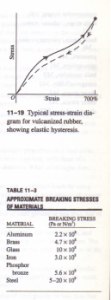# ELASTICITY AND PLASTICITY

11.8 ELASTICITY AND PLASTICITY

Hooke’s law, the proportionality of stress and strain in elastic deformations, has a limited range of validity. In the preceding three sections we used phrases such as “provided that the forces are small enough that Hooke’s law is obeyed.” Just what are the  imitations of Hooke’s law? We know that if you pull, squeeze, or twist anything hard enough, it will bend or break. Can we be more precise than that? Let’s look at tensile stress and strain again. Suppose we plot a graph of stress as a function of strain. If Hooke’s law is obeyed, the graph is a straight line with a slope equal to Young’s modulus. Figure 11-18 shows a typical stress-strain graph for a metal such as copper or soft iron. The strain is shown as the percent elongation; the horizontal scale is not uniform beyond the first portion of the curve, up to a strain of less than 1%. The first portion is a straight line, indicating Hooke’s law behavior with stress directly proportional to strain. This straight-line portion ends at point a; the stress at this point is called the proportional limit. From a to b, stress and strain are no longer proportional, and Hooke’s law is not obeyed. If the load is gradually removed, starting at any point between 0 and b, the curve is retraced until the material returns to its original length. The deformation is reversible, and the forces are conservative; the energy put into the material to cause the defoliation is recovered when the stress is removed. In region Ob we say that the material shows elastic behavior. Point b, the end of this region, is called the yield point; the stress at the yield point is called the elastic limit.When we increase the stress beyond point b, the strain continues to increase. But now when we remove the load at some point beyond b, say c, the material does not come back to its original length. Instead, it follows the red line in Fig. 11-18. The length at zero stress is now greater than the original length; the material has undergone an irreversible deformation and has acquired what we call a permanent set. Further increase of load beyond c produces a large increase in strain for a relatively small increase in stress, until a point d is reached at which fracture takes place. The behavior of the material from b to d is called plastic flow or plastic deformation. A plastic deformation is irreversible; when the stress is removed, the material does not return to its original state. For some materials a large amount of plastic deformation takes place between the elastic limit and the fracture point. Such a material is said to be ductile. But if fracture occurs soon after the elastic limit is passed, the material is said to be brittle. A soft iron wire that can have considerable permanent stretch without breaking is ductile, while a steel piano string that breaks soon after its elastic limit is reached is brittle. Figure 11-19 shows a stress-strain curve for vulcanized rubber that has been stretched by over seven times its original length. The stress is not proportional to the  strain, but the behavior is elastic because when the load is removed, the material returns to its original length. However, the material follows different curves for increasing and  decreasing stress. This is called elastic hysteresis. The work done by the material when · returns to its original shape is less than the work required to deform it; there are non conservative forces associated with internal friction. Rubber with large elastic hysteresis useful for absorbing vibrations, such as in engine mounts and shock-absorbed . gs for cars. 1be stress required to cause actual fracture of a material is called the breaking stress, !be ultimate strength, or (for tensile stress) the tensile strength. Two materials, such as types of steel, may have very similar elastic constants but vastly different breaking . Table 11-3 gives a few typical values of breaking stress for several materials in ‘OIL 1be conversion factor 6.9 x lOBPa = 100,000 psi will help put these numbers in perspective. For example, if the breaking stress of a particular steel is 6.9 x lOB Pa, then a with l-in.’ cross section has a breaking strength of 100,000 lb.